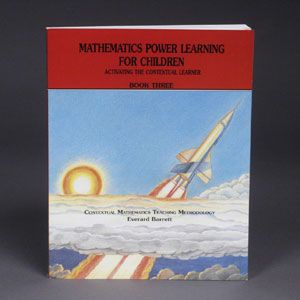Making You An Instant Master Teacher
•# Mathematics Power Learning Book 3 (6th-8th)

\$25.00

Power Learning Book 3 - For 5th through 7th graders and remediation of older learners. Original and effective methods for thorough understanding and mastery of multiplication/division of fractions, the decimal and metric systems, operations on decimals, transforming among fractions/decimals/percents and rounding off. Your learners should complete Book III in 10 months or less.

Additional item needed: Power Learning Workbook 3

SAMPLE LESSON

TELLING THE TRUTH ABOUT MULTIPLYING DECIMALS

Objective: The learners will find the product of any two decimals.

Write on the board: 7.3 x 4.23

Direct learners as follows:

"What fraction is equivalent to 7.3?" (73/10)
"What fraction is equivalent to 4.23?" (423/100)
"Can you multiply 73/10 by 423/100?"
"Do it!"
The learners' work will look like this (show on the board):"What decimal is equivalent to 30,879/1,000?" (30.879)
"So what is the answer to 7.3 x 4.23?"

The board work for the example above (7.3 x 4.23) should look like this:Write on the board:

4.7 x 3.6

Ask, "How will you do it?"

By means of the same questions in the first example, elicit the following as you write it on the board:Have learners do the following by means of the "fraction method" above.

 (a) .67 x 2.3 (b) 6.7 x .23 (c) .67 x .23 (d) 67 x .23 (e) 6.7 x 23 (f) .067 x .23 (g) 67 x .0023

Direct learners as follows:

"In the answers to examples (a) through (g), do you see the same set of digits?"
(1, 5, 4, 1)
"While doing each example, how did we get the digits 1, 5, 4, 1?" (In each example, we multiplied the numerators 67 and 23)

"In example (a), we multiplied a number, expressed as hundredths (.67), by a number expressed as tenths (2.3)."
"If we multiply hundredths by tenths, what do we get?" (Thousandths)
"So, how many decimal places must the answer have?" (Three)
"Hence, 67 hundredths times 23 tenths equal 1,541 thousandths." (1.541)

"In example (b), we multiplied a number, expressed as tenths (6.7), by a number expressed as hundredths (.23)."
"If we multiply tenths by hundredths, what do we get?"
"So, how many decimal places must the answer have?"
"Hence, 67 tenths times 23 hundredths equal 1,541 thousandths." (1.541)

"In example (c), we multiplied a number, expressed as hundredths (.67), by a number expressed as hundredths (.23)."
"If we multiply hundredths by hundredths, what do we get?" (ten-thousandths)
"So, how many decimal places must the answer have?" (Four)
"Hence, 67 hundredths times 23 hundredths equal 1,541 ten-thousandths." (.1541)

"In example (d), we multiplied a whole number (67) by a number expressed as hundredths (.23)."
"If we multiply a whole number by hundredths, what do we get?" (Hundredths)
"So, how many decimal places must the answer have?"
"Hence, 67 units times 23 hundredths equal 1,541 hundredths." (15.41)

"In example (e), we multiplied a number, expressed as tenths (6.7), by a whole number (23)."
"If we multiply tenths by a whole number, what do we get?"
"So, how many decimal places must the answer have?"
"Hence, 67 tenths times 23 units, equal 1,541 tenths." (154.1)

"In example (f), we multiplied a number, expressed as thousandths (.067), by a number expressed as hundredths (.23)."
"If we multiply thousandths by hundredths, what do we get?" (Hundred-thousandths)
"So, how many decimal places must the answer have?"
"Hence, 67 thousandths times 23 hundredths equal 1,541 hundred-thousandths." (.01541)

"In example (g), we multiplied a whole number (67) by a number expressed as ten-thousandths (.0023)."
"If we multiply a whole number by ten-thousandths what do we get?"
"So, how many decimal places must the answer have?"
"Hence, 67 units times 23 ten-thousandths equal 1,541 ten-thousandths." (.1541)

Write on the board:

.483 x 2.7

This example is not to be done by the fraction method.

Ask, "How will you find the digits in the answer to this multiplication?" (Multiply 483 by 27, since they are the numerators of the two fractions involved)

Write on the board:Direct learners as follows:

"Now we have the digits of the answer." (1, 3, 0, 4, 1)
"The problem, .483 x 2.7, multiplies thousandths by tenths. When we multiply thousandths by tenths, what do we get?"
"Since the answer to .483 x 2.7 is a decimal number in the ten-thousandths, how many decimal places are in the answer?"
"So, between which two digits of 13041 is the decimal point to be placed?" (Between 1 and 3)

Finally, learners conclude that:

.483 x 2.7 = 1.3041